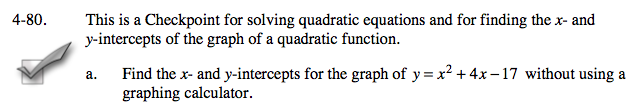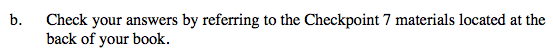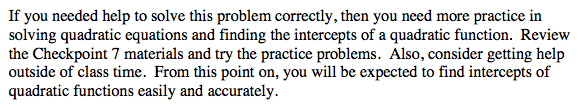### Home > A2C > Chapter 4 > Lesson 4.2.1 > Problem4-80

4-80.
1. This is a Checkpoint for solving quadratic equations and for finding the x- and y-intercepts of the graph of a quadratic function. Homework Help ✎If you needed help to solve this problem correctly, then you need more practice in solving quadratic equations and finding the intercepts of a quadratic function. Review the Checkpoint 7 materials and try the practice problems. Also, consider getting help outside of class time. From this point on, you will be expected to find intercepts of quadratic functions easily and accurately.

1. Find the x- and y-intercepts for the graph of y = x2 + 4x − 17 without using a graphing calculator.

2. Check your answers by referring to the Checkpoint 7 materials located at the back of your book.Answers and extra practice are located in the back of your printed textbook or in the Reference Tab of your eBook.
If you have an eBook for A2C , login and then click the following link: Checkpoint 7 Answers and Practice